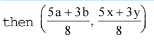# Coordinate Geometry - Olympiad Level MCQ, Class 10 Mathematics

## 25 Questions MCQ Test Olympiad Preparation for Class 10 | Coordinate Geometry - Olympiad Level MCQ, Class 10 Mathematics

Description
Attempt Coordinate Geometry - Olympiad Level MCQ, Class 10 Mathematics | 25 questions in 25 minutes | Mock test for Class 10 preparation | Free important questions MCQ to study Olympiad Preparation for Class 10 for Class 10 Exam | Download free PDF with solutions
QUESTION: 1

Solution:
QUESTION: 2

Solution:
QUESTION: 3

### The radius of the circle inscribed in the triangle formed by lines x = 0, y = 0, 4x + 3y – 24 = 0 is :

Solution:
QUESTION: 4

In a ΔABC, if A is the point (1, 2) and equations of the median through B and C are respectively x + y =

5 and x = 4, then B is :

Solution:
QUESTION: 5

The straight line 3x + y = 9 divides the segment joining the points (1, 3) and (2, 7) in the ratio :

Solution:
QUESTION: 6

Two opposite vertices of a rectangle are (1, 3) and (5, 1). If the equation of a diagonal of this rectangle

is y = 2x + c, then the value of c is :

Solution:
QUESTION: 7

The radius of the circle passing through the point (6, 2), two of whose diameters are x + y = 6 and x + 2y =

4 is :

Solution:
QUESTION: 8

The straight lines x + y = 0, 3x + y – 4 = 0, x + 3y – 4 = 0 form a triangle which is :

Solution:
QUESTION: 9

lines segment joining the points (1, 2) and (– 2, 1) is divided by the line 3x + 4y = 7 in the ratio :

Solution:
QUESTION: 10

If a, b, c are in A.P. then the straight line ax + by + c = 0 will always pass through a fixed point whose

co-ordinates are :

Solution:
QUESTION: 11

The lines 8x + 4y = 1, 8x + 4y = 5, 4x + 8y = 3, 4x + 8y = 7 form a :

Solution:
QUESTION: 12

The incentre of the triangle formed by the lines y = 15, 12y = 5x and 3x + 4y = 0 is :

Solution:
QUESTION: 13

The area of triangle formed by the lines y = x, y = 2x and y = 3x + 4 is :

Solution:
QUESTION: 14

The triangle formed by the lines x + y = 1, 2x + 3y – 6 = 0 and 4x – y + 4 = 0 lies in the :

Solution:
QUESTION: 15

A line is drawn through the points (3, 4) and (5, 6). If the line is extended to a point whose ordinate is – 1,

then the abscissa of that point is :

Solution:
QUESTION: 16

The area of the triangle whose sides are along the lines x = 0, y = 0 and 4x + 5y = 20 is :

Solution:
QUESTION: 17

If a, b, c are all distinct, then the equations (b – c) x + (c – a) y + a – b = 0 and (b3 – c3) x + (c3 – a3) y+ a3 – b3 = 0 represent the same line if :

Solution:
QUESTION: 18

The area of the quadrilateral with vertices at (4, 3), (2, – 1), (– 1, 2), (– 3, – 2) is :

Solution:
QUESTION: 19

If α,β,γ are the real roots of the equation x3 – 3px2 + 3qx – 1 = 0, then the centroid of the triangle with

vertices (α,1/α),(β,1/β)  and (γ,1/β) is at the point :

Solution:
QUESTION: 20

The co-ordinates of A, B, C are (6, 3), (– 3, 5), (4, – 2) respectively and P is any point (x, y). The ratio of

the areas of ΔPBC and ΔABC is :

Solution:
QUESTION: 21

The area of a triangle is 5 square units. Two of its vertices are (2, 1) and (3, – 2). The third vertex

lie on y = x + 3, the third vertex is :(7/2,)

Solution:
QUESTION: 22

The point of intersection of the lines x/a+y/b=1 and x/b+y/a=1, lies on the line :

Solution:
QUESTION: 23

The point A divides the join of the points (–5, 1) and (3, 5) in the ratio k : 1 and co-ordinates of points B

and C are (1, 5) and (7, – 2) respectively. If the area of ΔABC be 2 units, then k equals :

Solution:
QUESTION: 24

Q,R and S are the points on the line joining the points P(a, x) and T(b,y) such that PQ = QR = RS = ST,is the mid point of the segment :

Solution:
QUESTION: 25

The triangle with vertices A(2, 7), B(4, y) and C(–2, 6) is right angled at A if :

Solution:Use Code STAYHOME200 and get INR 200 additional OFF Use Coupon Code